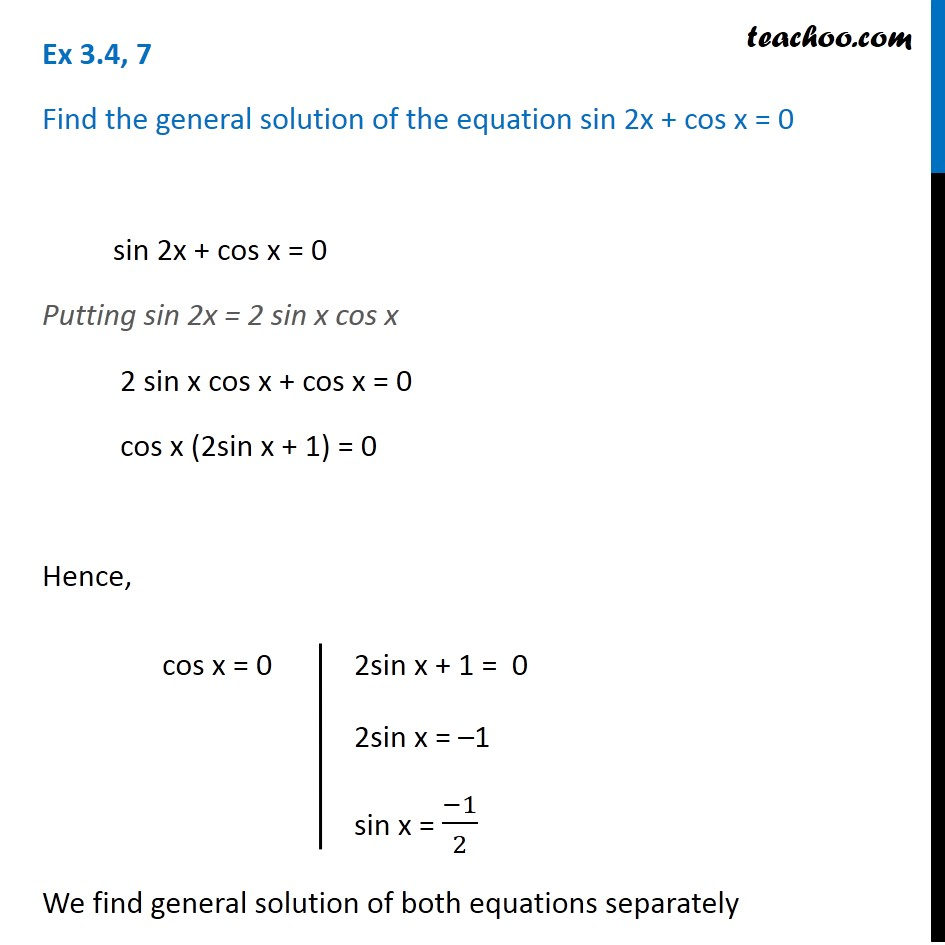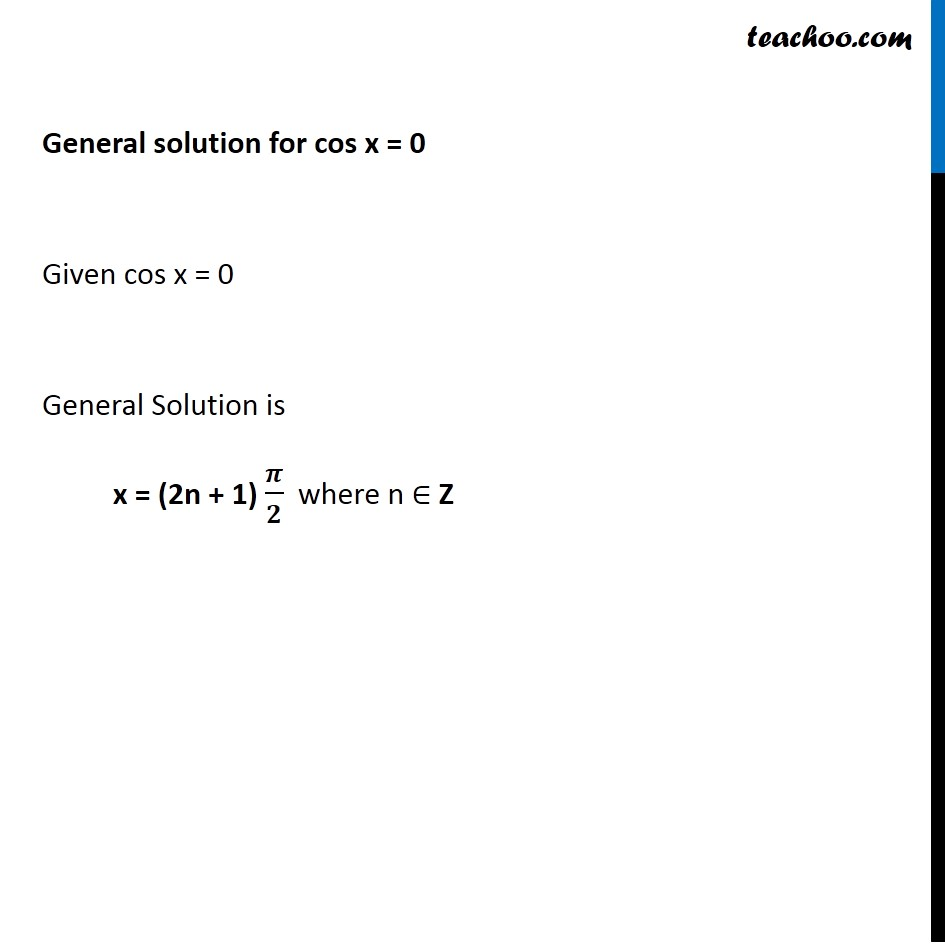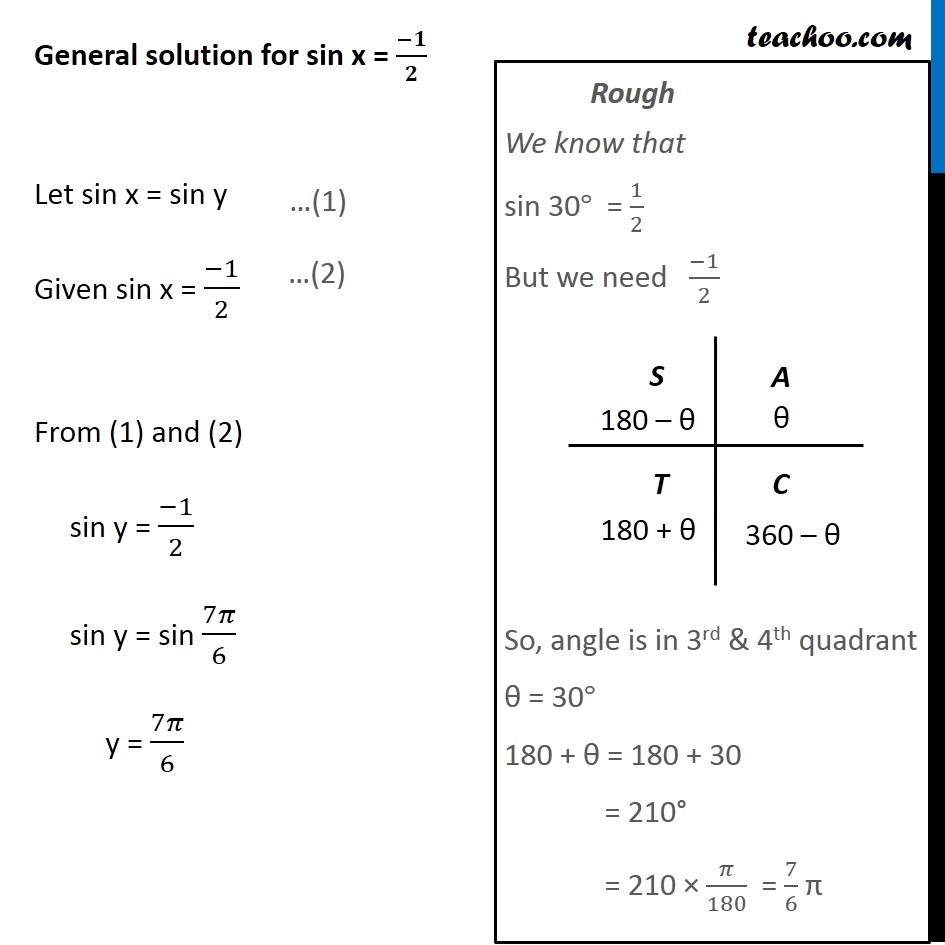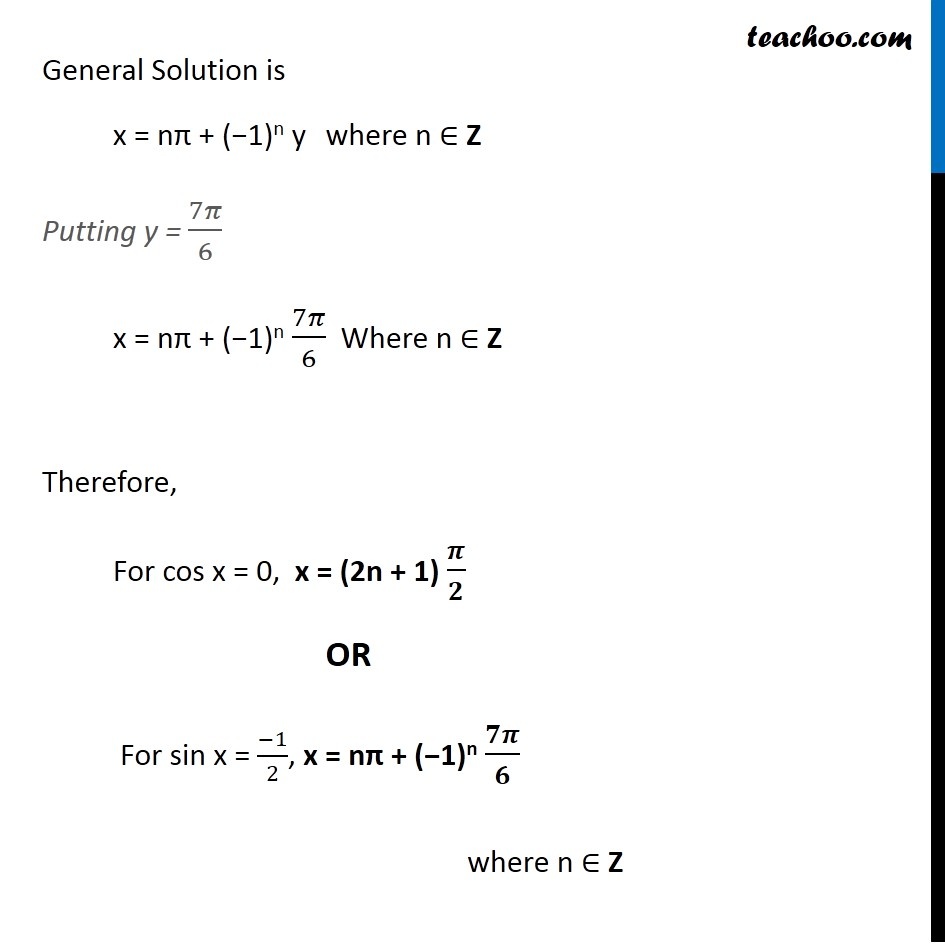Principal and General Solutions

Chapter 3 Class 11 Trigonometric Functions
Serial order wiseLearn in your speed, with individual attention - Teachoo Maths 1-on-1 Class

### Transcript

Question 7 Find the general solution of the equation sin 2x + cos x = 0 sin 2x + cos x = 0 Putting sin 2x = 2 sin x cos x 2 sin x cos x + cos x = 0 cos x (2sin x + 1) = 0 Hence, We find general solution of both equations separately cos x = 0 2sin x + 1 = 0 2sin x = –1 sin x = (−1)/2 General solution for cos x = 0 Given cos x = 0 General Solution is x = (2n + 1) 𝝅/𝟐 where n ∈ Z General solution for sin x = (−𝟏)/𝟐 Let sin x = sin y Given sin x = (−1)/2 From (1) and (2) sin y = (−1)/2 sin y = sin 7𝜋/6 y = 7𝜋/6 Rough We know that sin 30° = 1/2 But we need (−1)/2 So, angle is in 3rd & 4th quadrant θ = 30° 180 + θ = 180 + 30 = 210° = 210 × 𝜋/180 = 7/6 π General Solution is x = nπ + (−1)n y where n ∈ Z Putting y = 7𝜋/6 x = nπ + (−1)n 7𝜋/6 Where n ∈ Z Therefore, For cos x = 0, x = (2n + 1) 𝝅/𝟐 OR For sin x = (−1)/2, x = nπ + (−1)n 𝟕𝝅/𝟔 where n ∈ Z